# Co-vsCounter-CurrentExchange

Model number
0129

Steady-state air-blood exchange for two geometric configurations.

## Description

``` Steady-state air blood exchange is presented for two different hypothetical geometric
configurations, i.e., for co-current and counter-current air-blood exchange.
Alveoli-capillary interconnection is modeled as two co-centric cylinders with air and
blood flowing in the same direction for co-current flow and in the opposite direction
in the counter-current flow.

```

## Equations

If diffusion and reaction is neglected the equations of the system can be written in the following form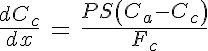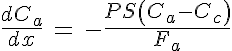where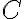is the concentration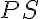is the permeability-surface product,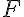is the volumetric flow rate,is the non-dimensional spatial coordinate,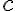is the index referring to capillary, and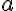is the index of alveoli.

The set of ODE can be solved as an initial value problem in case of co-current flow and as a boundary value problem in case of counter-current flow. Accordingly, the following analytical solutions can be obtain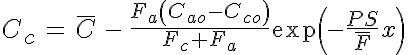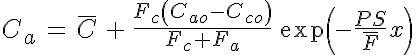in case of co-current flow and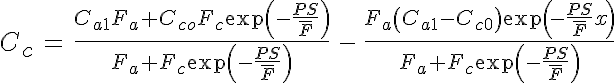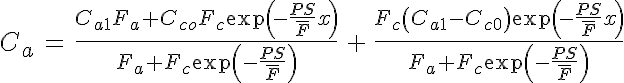in case of counter-current flow, where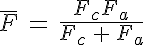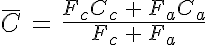the secondary subscripts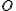and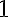refers to the conditions imposed at the opposite ends of the cylinder. Integration of the differenceover the length of the cylinder yields the total mass exchange rate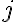from air to blood. Thus, the following relations between mass exchange rate and permeability-surface product can be obtained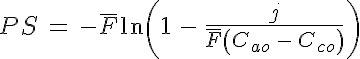for co-current flow and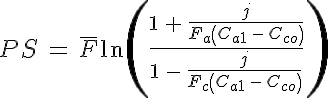The equations for this model may be viewed by running the JSim model applet and clicking on the Source tab at the bottom left of JSim's Run Time graphical user interface. The equations are written in JSim's Mathematical Modeling Language (MML). See the Introduction to MML and the MML Reference Manual. Additional documentation for MML can be found by using the search option at the Physiome home page.

References

None.

Key terms
co-current
counter-current